# Math > Year 2

## Comparing and Sequencing intervals of time

Interval
A space or gap between two numbers.

Time intervals
A specific period of time spent on any event. We can tell the time interval by using the start and end times of an event.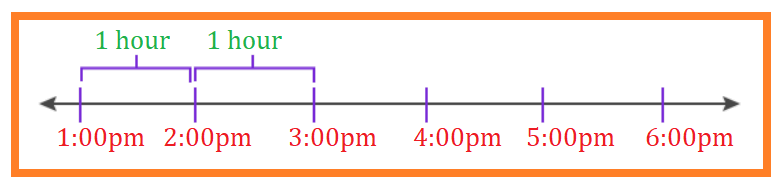The time interval between 1: 00 pm and 3:00 pm is 2 hours.

How to compare the intervals of time?

We will use the number line to compare the intervals of time.

Let’s take a look at the example given below.

Shay studied from 10:30 A.M. to 2:30 P.M.

He went to a party at 6: 30 P.M. and came back at 9:30 P.M.  Did Shay spend more time studying or at the party?

Explanation:

We can plot the time on the number line and then we can find the time intervals.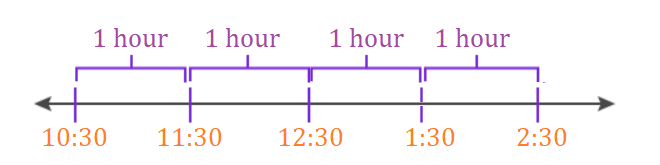The time interval between 10: 30 A.M. and 2: 30 P.M. is 4 hours.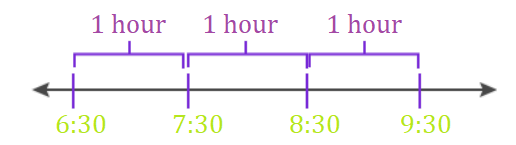The time interval between 6: 30 and 9:30 are 3 hours.

Shay spent more time on study i.e.  4 hours.

Sequencing intervals of time:

We can order the time either from longest to shortest duration or from shortest to longest duration.

Example 1:

Sequence the following durations from shortest to longest.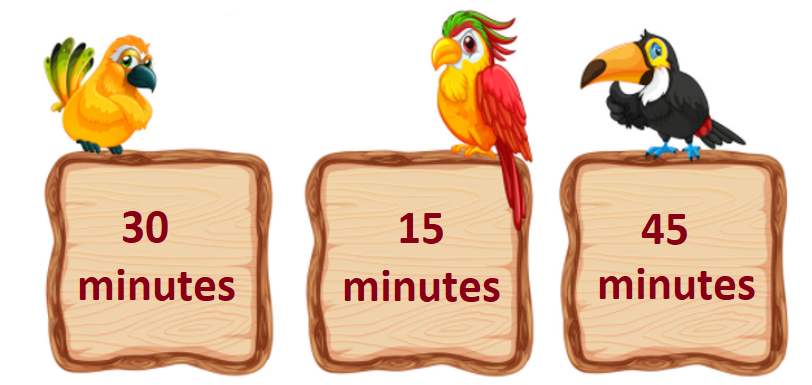While sequencing we need to observe the units properly.

As we can see all the durations are given in minutes we can simply arrange them from the shortest to the longest.

15 minutes, 30 minutes, 45 minutes

Example 2:

Compare the given time durations.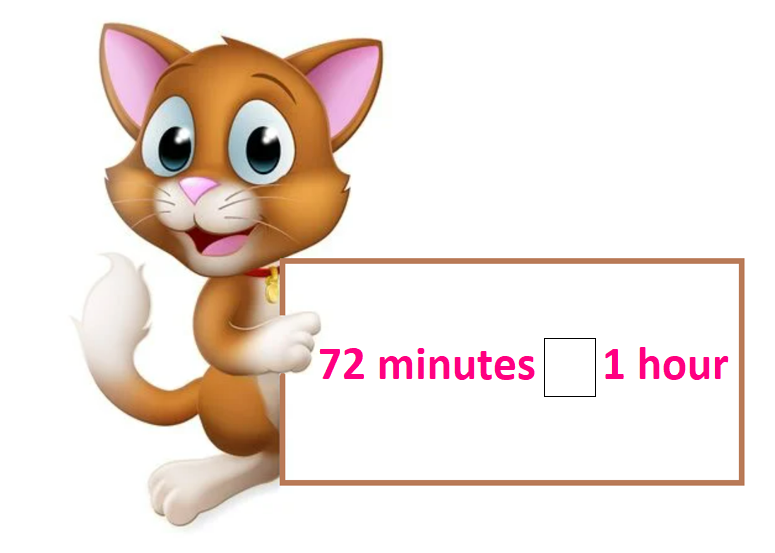We can see that both the units are different.

We can make the units the same.

1 hour = 60 minutes.

72 minutes > 60 minutes

72 minutes > 1 hour

//